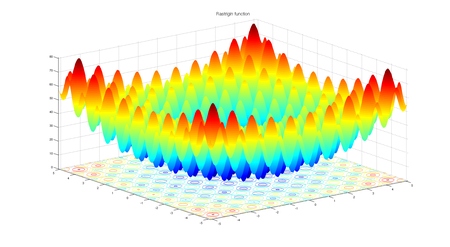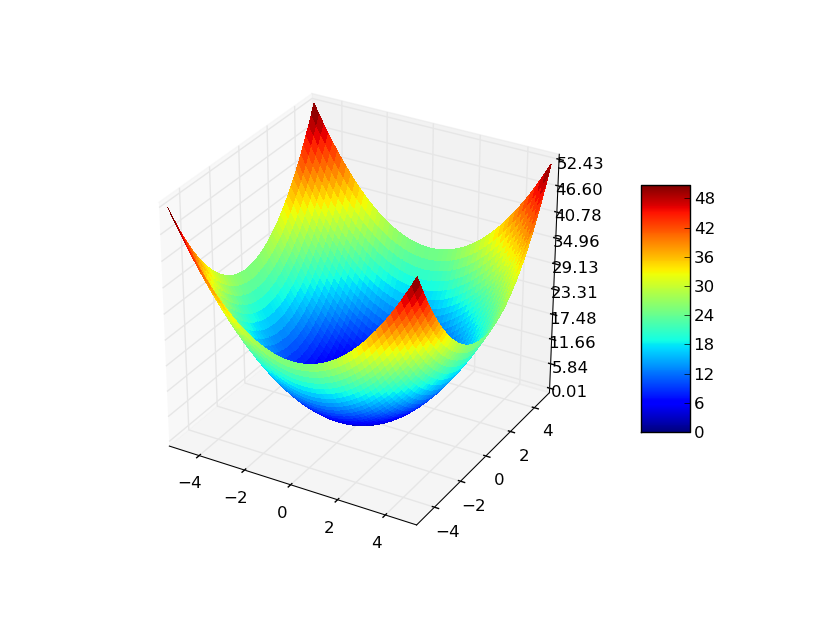# Implementation of Whale Optimization Algorithm

• Last Updated : 13 Dec, 2021

Previous article Whale optimization algorithm (WOA) talked about the inspiration of whale optimization, its mathematical modeling and algorithm. In this article we will implement a whale optimization algorithm (WOA) for two fitness functions 1) Rastrigin function    2) Sphere function  The algorithm will run for a predefined number of maximum iterations and will try to find the minimum value of these fitness functions.

## Fitness functions

### 1) Rastrigin function

Rastrigin function is a non-convex function and is often used as a performance test problem for optimization algorithms.

#### function equation:Fig1: Rastrigin function for 2 variables

For an optimization algorithm, rastrigin function is a very challenging one. Its complex behavior causes optimization algorithms to often be stuck at local minima. Having a lot of cosine oscillations on the plane introduces the complex behavior to this function.

### 2) Sphere function

Sphere function is a standard function for evaluating the performance of an optimization algorithm.

#### function equation:Fig 2: Sphere function for 2 variables

## Choice of hyper-parameters

### Parameters of problem:

• Number of dimensions (d) = 3
• Lower bound (minx) = -10.0
• Upper bound (maxx) = 10.0

### Hyperparameters of the algorithm:

• Number of particles (N) = 50
• Maximum number of iterations (max_iter) = 100
• spiral coefficient (b) = 1

### Inputs

• Fitness function
• Problem parameters ( mentioned above)
• Population size (N) and Maximum number of iterations  (max_iter)
• Algorithm Specific hyperparameter b

## Algorithm

The algorithm of the whale optimization and mathematical equations are already described in the previous article

## Python3

 # python implementation of whale optimization algorithm (WOA)# minimizing rastrigin and sphere function  import randomimport math  # cos() for Rastriginimport copy  # array-copying convenienceimport sys  # max float  # -------fitness functions--------- # rastrigin functiondef fitness_rastrigin(position):    fitness_value = 0.0    for i in range(len(position)):        xi = position[i]        fitness_value += (xi * xi) - (10 * math.cos(2 * math.pi * xi)) + 10    return fitness_value  # sphere functiondef fitness_sphere(position):    fitness_value = 0.0    for i in range(len(position)):        xi = position[i]        fitness_value += (xi * xi);    return fitness_value;  # -------------------------  # whale classclass whale:    def __init__(self, fitness, dim, minx, maxx, seed):        self.rnd = random.Random(seed)        self.position = [0.0 for i in range(dim)]         for i in range(dim):            self.position[i] = ((maxx - minx) * self.rnd.random() + minx)         self.fitness = fitness(self.position)  # curr fitness  # whale optimization algorithm(WOA)def woa(fitness, max_iter, n, dim, minx, maxx):    rnd = random.Random(0)     # create n random whales    whalePopulation = [whale(fitness, dim, minx, maxx, i) for i in range(n)]     # compute the value of best_position and best_fitness in the whale Population    Xbest = [0.0 for i in range(dim)]    Fbest = sys.float_info.max     for i in range(n):  # check each whale        if whalePopulation[i].fitness < Fbest:            Fbest = whalePopulation[i].fitness            Xbest = copy.copy(whalePopulation[i].position)     # main loop of woa    Iter = 0    while Iter < max_iter:         # after every 10 iterations        # print iteration number and best fitness value so far        if Iter % 10 == 0 and Iter > 1:            print("Iter = " + str(Iter) + " best fitness = %.3f" % Fbest)         # linearly decreased from 2 to 0        a = 2 * (1 - Iter / max_iter)        a2=-1+Iter*((-1)/max_iter)         for i in range(n):            A = 2 * a * rnd.random() - a            C = 2 * rnd.random()            b = 1            l = (a2-1)*rnd.random()+1;            p = rnd.random()             D = [0.0 for i in range(dim)]            D1 = [0.0 for i in range(dim)]            Xnew = [0.0 for i in range(dim)]            Xrand = [0.0 for i in range(dim)]            if p < 0.5:                if abs(A) > 1:                    for j in range(dim):                        D[j] = abs(C * Xbest[j] - whalePopulation[i].position[j])                        Xnew[j] = Xbest[j] - A * D[j]                else:                    p = random.randint(0, n - 1)                    while (p == i):                        p = random.randint(0, n - 1)                     Xrand = whalePopulation[p].position                     for j in range(dim):                        D[j] = abs(C * Xrand[j] - whalePopulation[i].position[j])                        Xnew[j] = Xrand[j] - A * D[j]            else:                for j in range(dim):                    D1[j] = abs(Xbest[j] - whalePopulation[i].position[j])                    Xnew[j] = D1[j] * math.exp(b * l) * math.cos(2 * math.pi * l) + Xbest[j]             for j in range(dim):                whalePopulation[i].position[j] = Xnew[j]         for i in range(n):            # if Xnew < minx OR Xnew > maxx            # then clip it            for j in range(dim):                whalePopulation[i].position[j] = max(whalePopulation[i].position[j], minx)                whalePopulation[i].position[j] = min(whalePopulation[i].position[j], maxx)             whalePopulation[i].fitness = fitness(whalePopulation[i].position)             if (whalePopulation[i].fitness < Fbest):                Xbest = copy.copy(whalePopulation[i].position)                Fbest = whalePopulation[i].fitness          Iter += 1    # end-while     # returning the best solution    return Xbest  # ----------------------------  # Driver code for rastrigin function print("\nBegin whale optimization algorithm on rastrigin function\n")dim = 3fitness = fitness_rastrigin print("Goal is to minimize Rastrigin's function in " + str(dim) + " variables")print("Function has known min = 0.0 at (", end="")for i in range(dim - 1):    print("0, ", end="")print("0)") num_whales = 50max_iter = 100 print("Setting num_whales = " + str(num_whales))print("Setting max_iter    = " + str(max_iter))print("\nStarting WOA algorithm\n") best_position = woa(fitness, max_iter, num_whales, dim, -10.0, 10.0) print("\nWOA completed\n")print("\nBest solution found:")print(["%.6f" % best_position[k] for k in range(dim)])err = fitness(best_position)print("fitness of best solution = %.6f" % err) print("\nEnd WOA for rastrigin\n") print()print() # Driver code for Sphere functionprint("\nBegin whale optimization algorithm on sphere function\n")dim = 3fitness = fitness_sphere print("Goal is to minimize sphere function in " + str(dim) + " variables")print("Function has known min = 0.0 at (", end="")for i in range(dim - 1):    print("0, ", end="")print("0)") num_whales = 50max_iter = 100 print("Setting num_whales = " + str(num_whales))print("Setting max_iter    = " + str(max_iter))print("\nStarting WOA algorithm\n") best_position = woa(fitness, max_iter, num_whales, dim, -10.0, 10.0) print("\nWOA completed\n")print("\nBest solution found:")print(["%.6f" % best_position[k] for k in range(dim)])err = fitness(best_position)print("fitness of best solution = %.6f" % err) print("\nEnd WOA for sphere\n")

## Output

Begin whale optimization algorithm on rastrigin function

Goal is to minimize Rastrigin's function in 3 variables
Function has known min = 0.0 at (0, 0, 0)
Setting num_whales = 50
Setting max_iter    = 100

Starting WOA algorithm

Iter = 10 best fitness = 0.018
Iter = 20 best fitness = 0.000
Iter = 30 best fitness = 0.000
Iter = 40 best fitness = 0.000
Iter = 50 best fitness = 0.000
Iter = 60 best fitness = 0.000
Iter = 70 best fitness = 0.000
Iter = 80 best fitness = 0.000
Iter = 90 best fitness = 0.000

WOA completed

Best solution found:
['0.000000', '-0.000000', '-0.000000']
fitness of best solution = 0.000000

End WOA for rastrigin

Begin whale optimization algorithm on sphere function

Goal is to minimize sphere function in 3 variables
Function has known min = 0.0 at (0, 0, 0)
Setting num_whales = 50
Setting max_iter    = 100

Starting WOA algorithm

Iter = 10 best fitness = 0.130
Iter = 20 best fitness = 0.000
Iter = 30 best fitness = 0.000
Iter = 40 best fitness = 0.000
Iter = 50 best fitness = 0.000
Iter = 60 best fitness = 0.000
Iter = 70 best fitness = 0.000
Iter = 80 best fitness = 0.000
Iter = 90 best fitness = 0.000

WOA completed

Best solution found:
['0.000000', '0.000000', '-0.000000']
fitness of best solution = 0.000000

End WOA for sphere

### References:

Research paper: https://www.sciencedirect.com/science/article/pii/S0965997816300163

Author’s original implementation (in MATLAB): https://www.mathworks.com/matlabcentral/fileexchange/55667-the-whale-optimization-algorithm

My Personal Notes arrow_drop_up